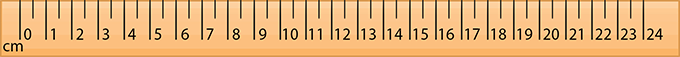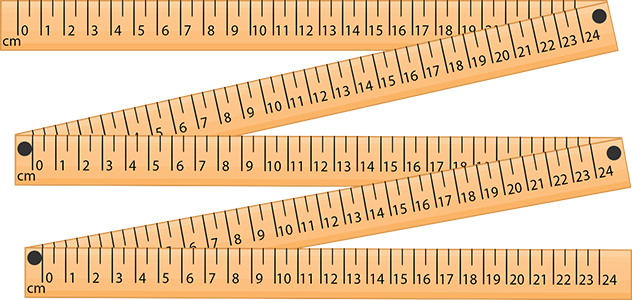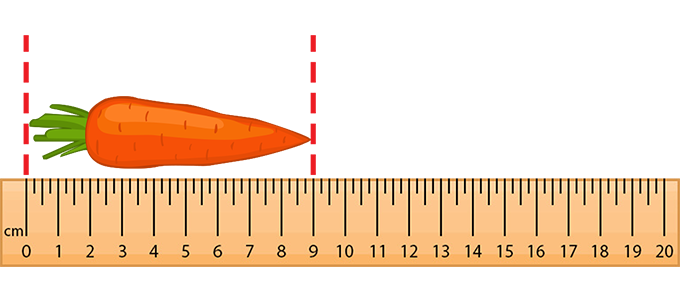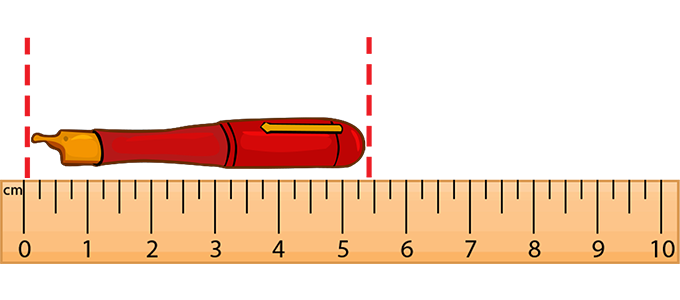# Centimeter - Definition with Examples

The Complete K-5 Math Learning Program Built for Your Child

• 30 Million Kids

Loved by kids and parent worldwide

• 50,000 Schools

Trusted by teachers across schools

• Comprehensive Curriculum

Aligned to Common Core

What is a Centimeter?

A centimeter is a metric unit of measurement used for measuring the length of an object. It is written as cm. The three standard tools of measurement are:

 1. Ruler : A ruler is the most common measurement tool in mathematics. It is used to measure small objects, for example, notebooks, pencils, and bottles. Most rulers come with two measurement metrics, centimeters, and inches and are made usually in lengths of 15 cm and 30 cm.2. Meter stick : A meter stick is a measurement tool that measures one meter (hundred centimeters) and is used to measure things in meters and centimeters. Things like the length of a table or the width of a bag can be measured using a meter stick.Here are some things that can be measured in centimeters:

• The waist size of a pair of trousers
• Length of the fish caught
• The radius of a car’s tires
• The distance between a person’s eyes

Relation to other standard units:

1. A meter has 100 centimeters.
2. 10 millimeters make one centimeter.
3. The centimeter can be written as cm.
4. While calculating the surface area of an object, the unit of measurement becomes cm2.
5. While measuring the volume of an object, the unit of measurement becomes cm3Fun facts about centimeters: The word “centimeter” is a combination of the Latin word centum, which means hundred, and the French word mètre. The word centimeter was first coined in the year 1801 and has been widely used since then.

Won Numerous Awards & Honors